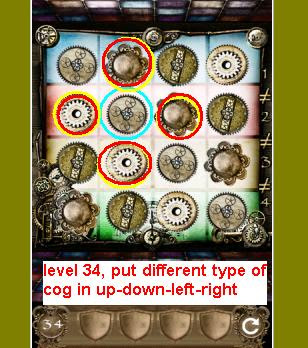100 Gates Level 35

This is really difficult game because it's math :) but be calm we have got the answer for 100 gates level 35 and 34. please read our hintsGame Stage 34
Well this on is not math puzzle , so it's not difficult
You only have to arrange cog with no same type of cog around one type of cog
The strategy is like minesweaper game, put one cog then put other four cogs around with different type cog (diagonal is fine), look at our sample correct arrangement

"100 Gates Level 35" game
You have to find missing number in this level
First Clean the screen from leaves by shaking your smartphone.
Now you can see clearly the number puzzles, There are 3 puzzle
For above the door puzzle,  it's actually fibonacci sequence, if you forget this math equation please open your school book :) forget it simply add every 2 number in the array, look at this sequence : 1+2 =3 then 2+3=5 then 3+5=8 so the answer for the puzzle is 8
Other missing number are 9 and 3

100 gates 35so the solution for this game is

• tap top left skull by 8x
• tap bottom left skull by 9x
• tap bottom right skull by 3x

We have finished 100 gates level 35 game. so please look at the next guide here